# How does a PLL work?

Summary:
I'm struggling to understand how PLL works once the frequency is locked.
From reference:
https://www.elprocus.com/phase-locked-loop-operating-principle-and-applications/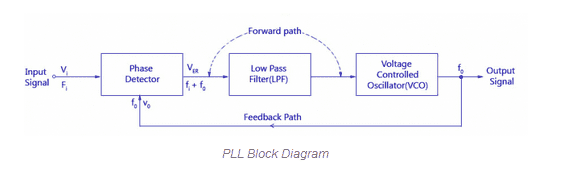It says that the PD produces an output that is DC level proportional to (Fi - Fo) (I think it has typo. It should be '-' but they've printed '+').
Then the low pass filter removes the high frequency noise and then we give this voltage difference to a VCO to get output frequency proportional to the phase difference.
But then what?
Suppose Fi is 50 Hz. Initially Fo is 0Hz. So output of phase detector is a signal proportional to Fi - Fo which is 50 Hz. So the VCO will produce an output which is proportional to (50 Hz - 0 Hz) = 50 Hz.
Then this becomes Fo = 50 Hz. So now Fi - Fo becomes 50 - 50 = 0Hz. So VCO will get 0Hz and will produce output proportional 0 Hz?

As quoted in the website:
Block Diagram And Working Principle Of PLL

The phase locked loop consists of a phase detector, a voltage control oscillator and, in between them, a low pass filter is fixed. The input signal ‘Vi’ with an input frequency ‘Fi’ is conceded by a phase detector. Basically the phase detector is a comparator which compares the input frequency fi through the feedback frequency fo. The output of the phase detector is (fi+fo) which is a DC voltage. The out of the phase detector, i.e., DC voltage is input to the low pass filter (LPF); it removes the high frequency noise and produces a steady DC level, i.e., Fi-Fo. The Vf is also a dynamic characteristic of the PLL.

The output of the low pass filter, i.e., DC level is passed on to the VCO. The input signal is directly proportional to the output frequency of the VCO (fo). The input and output frequencies are compared and adjusted through the feedback loop until the output frequency is equal to the input frequency. Hence, the PLL works like free running, capture, and phase lock.

When there is no input voltage applied, then it is said to be as a free running stage. As soon as the input frequency applied to the VOC changes and produces an output frequency for comparison, it is called as capture stage. The below figure shows the block diagram of the PLL.

Phase Locked Loop Detector

The phase locked loop detector compares the input frequency and the output frequency of the VCO to produces a DC voltage which is directly proportional to the phase distinction of the two frequencies.

Tom.G
Summary: I'm struggling to understand how PLL works once the frequency is locked.

It says that the PD produces an output that is DC level proportional to (Fi - Fo) (I think it has typo. It should be '-' but they've printed '+').
Not just a typo, it's a wrong definition.

The Phase Detector detects the instantaneous Phase Difference between the two signals. This of course means that when the input frequencies are different, the output varies on each input cycle. The Low Pass Filter averages this output and sends an almost-DC voltage to the VCO to change the VCO frequency to match the input frequency.

As the two frequencies approach each other, the output of the Low Pass Filter settles towards a constant DC value, and the VCO settles to a frequency that matches the input.

Here is a image that shows one implementation of a Phase Detector. It has an output when the two inputs are different.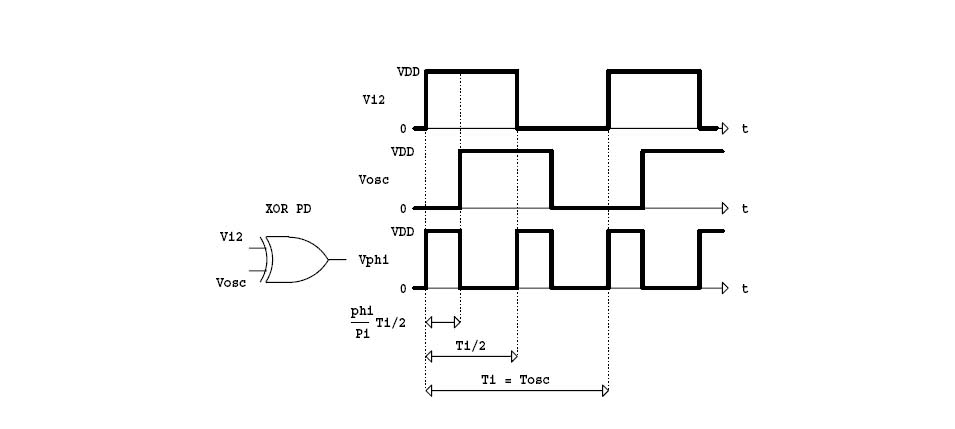Cheers,
Tom

•davenn and jaus tail
Not just a typo, it's a wrong definition.

The Phase Detector detects the instantaneous Phase Difference between the two signals. This of course means that when the input frequencies are different, the output varies on each input cycle. The Low Pass Filter averages this output and sends an almost-DC voltage to the VCO to change the VCO frequency to match the input frequency.

As the two frequencies approach each other, the output of the Low Pass Filter settles towards a constant DC value, and the VCO settles to a frequency that matches the input.

Here is a image that shows one implementation of a Phase Detector. It has an output when the two inputs are different.
View attachment 251522

Cheers,
Tom
So once the output frequency matches the input frequency, will the phase detector give any output signal or will it give zero?
Let's assume at some point difference between input phase and feedback phase is 30 degrees. So VCO will get a DC signal that is proportional to 30 degrees. So VCO will produce an output signal with some frequency. Now what will happen? Will the phase difference of 30 degrees reduce with each cycle?

Svein
So once the output frequency matches the input frequency, will the phase detector give any output signal or will it give zero?
The output signal of the phase detector shown above is just a digital signal. The low-pass filter converts it into an analog signal: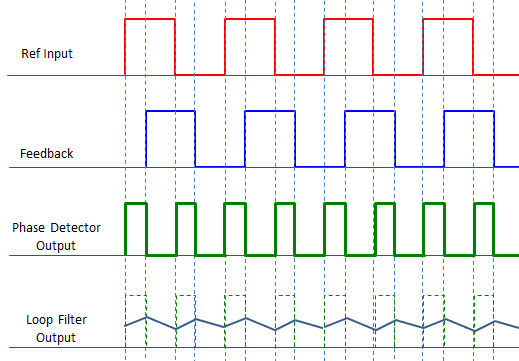Be aware that this simple phase detector only works for "small" frequency deviations. It will give the same output for all harmonics of the "ref. input".

••jaus tail and anorlunda
So if the output frequency is same as input frequency why would the PLL be used? To get stable frequency as output?

davenn
Gold Member
So if the output frequency is same as input frequency why would the PLL be used? To get stable frequency as output?

Yes

That's the whole point, it is to get a stable output
No oscillator is perfectly stable, this is one way of improving it
Some of the things that can vary the results, phase noise, reference oscillator stability, stray capacitance and inductance between components ( hence why in many PLL circuits, wax is poured over them to give mechanical stability) and temperature

•jaus tail
Tom.G
So once the output frequency matches the input frequency, will the phase detector give any output signal or will it give zero?
That depends on the particular Phase Detector (PD) design and on what the VCO needs.

In the usual simplest case, the PD will put out 1/2 the maximum control voltage when the two equal-frequency input signals are 90° out of phase. The design procedure then is to use component values for the Oscillator so the desired frequency is generated with 1/2 the maximum control voltage. This allows the widest oscillator frequency range above and below the input signal frequency.

Cheers,
Tom

•jaus tail
Averagesupernova
Gold Member
A PLL system can boast the flexibility of synthesizing many different frequencies. This is the main advantage of a PLL system. A variable frequency divider between the output and the phase detector allows the output frequency to be stepped at a frequency that is equal to the frequency that the phase detector is operating at. There are many many schemes used to make a flexible synthesizer that can step in smaller increments. Imagination is the limit. With direct digital synthesis, phase locked loop has somewhat gone out of style.

Last edited:
•jaus tail
I'm just having a hard time to understand why convert phase difference to frequency output. I mean in feedback the goal is to reduce output error.
Like you run a system, and the feedback will give part of output to the input. This will be error and the final goal is to reduce error by using feedback. This seems to be missing here as when I'm generating a frequency output, there is still a phase difference between output and input.

Baluncore
This seems to be missing here as when I'm generating a frequency output, there is still a phase difference between output and input.
Two signals locked with a PLL will have the same frequency, but they can have slightly shifted phases. The phase detector topology and the gain of the feedback loop will determine the phase difference when the VCO output is locked to the input signal.

The VCO search for, and the final capture of the locked condition, is a statistical process and cannot be easily predicted.

I have never found a real PLL that would not lock, but I still have a recurring nightmare that features a PLL that will dance around but never lock.

•jaus tail
anorlunda
Staff Emeritus
I'm just having a hard time to understand why convert phase difference to frequency output. I mean in feedback the goal is to reduce output error.
Have you considered the difficulty of measuring frequency, especially when the measuring period is less than a full cycle?

Two signals with different frequencies, produce a time varying phase difference. I can detect that, and infer a frequency difference even if I don't know exactly what the two frequencies are.

Saying it another way, you don't have 2 frequency sensors and a summer to calculate the error. You have a single frequency difference sensor.

Here's an analogy. In power we use an instrument called a synchroscope. It displays phase difference between two signals. If the frequencies of the two differ, the needle rotates one way or the other. So if the needle is not moving, you know that the frequencies are the same, but you don't know the values of the two frequencies. In this case we want both the frequency difference and the relative phase of the two signals to be zero before closing the circuit breaker.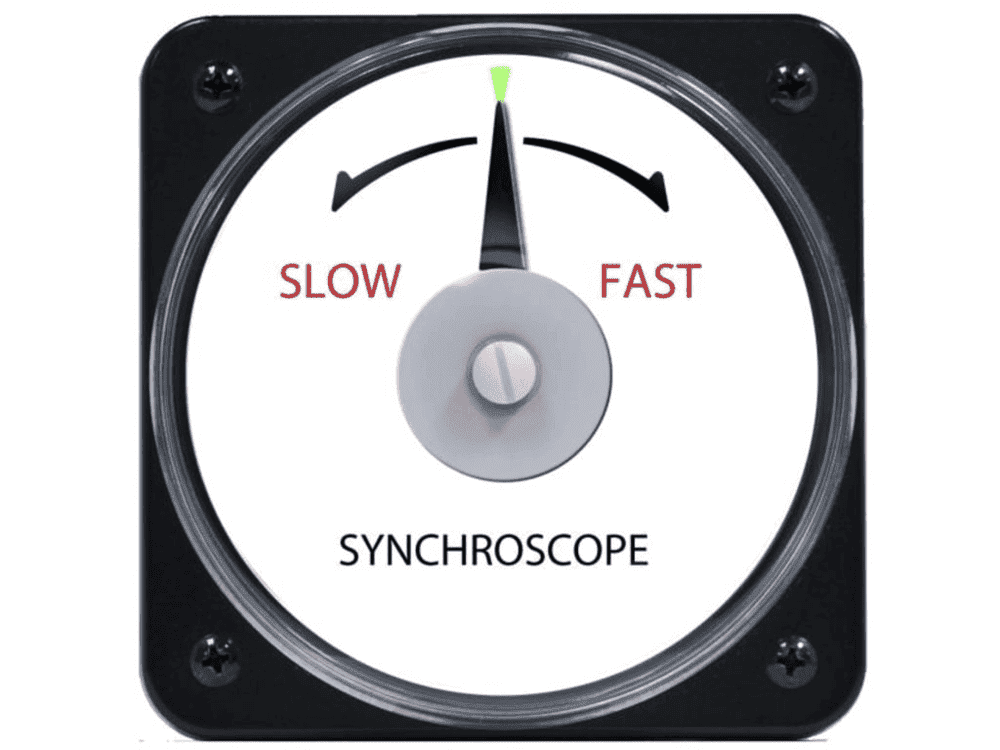•jaus tail
So if the output frequency is same as input frequency why would the PLL be used? To get stable frequency as output?

No - two frequencies from two different sources are NEVER identical! Therefore, you need a locked PLL to make these frequencies equal - and locked in phase!

•jaus tail
Averagesupernova
Gold Member
I'm just having a hard time to understand why convert phase difference to frequency output. I mean in feedback the goal is to reduce output error.
Like you run a system, and the feedback will give part of output to the input. This will be error and the final goal is to reduce error by using feedback. This seems to be missing here as when I'm generating a frequency output, there is still a phase difference between output and input.
The goal of PLL in most cases is control of an oscillator with digital accuracy. The goal is NOT to increase the accuracy over what the reference is. The output of a PLL synthesizer is NEVER more stable or accurate than the reference.
-
In PLL sythesizers in a radio system for example, suppose we want to be able to tune a local oscillator for the AM broadcast band. This would typically involve an oscillator that has a range from 995 KHz to 2055 KHz. The goal is to have an oscillator that will tune in appropriate steps within that range. We don't really care about the output of the phase detector other than using it to tell the VCO to tune up or down. Of course this is a simplified point of view.
-
In your diagram in the post#1 in what is labeled as the feedback path, insert a frequency divider that is variable for 199 to 411. Set the reference frequency at 5 KHz. Now do the math and you will determine that you can vary the frequency of the VCO in 5 KHz steps throughout the 995 KHz to 2055 KHz range by changing the divide rate.
-
Edit: By reference frequency I mean what is referred to as the input signal in your diagram.

Last edited:
•jaus tail
Averagesupernova
Gold Member
I have never found a real PLL that would not lock, but I still have a recurring nightmare that features a PLL that will dance around but never lock.
I lived your nightmare for a significant number of years troubleshooting communications systems PLLs. I believe I posted this another time here on PF, but a wise engineer once clued me in about how to troubleshoot an unruly phase locked loop. Break the loop. Control the VCO manually and watch the filtered phase detector output. Any time it would flop about around the intended frequency but not settle in stable-like it indicated a loop filter problem or possibly noise getting into the VCO. Watching the output of the VCO controlled manually with a stable voltage can indicate issues as well. Other than slow drift, is should be as stable as when locked.

••jaus tail and Baluncore
I'm just having a hard time to understand why convert phase difference to frequency output. I mean in feedback the goal is to reduce output error.

The goal of the control is to make the phase difference between input and output zero. It does it by measuring the phase difference between two signal now. If it finds a difference in this edge placement, it will either need to lengthen or shorten the time (a.k.a. phase) when the next edge happens to cancel out that difference. But changing where an edge happens is the same as changing its period, which is also changing its frequency. Therefore a PLL control system measures phase for its sensor, but uses frequency as the output of the control system’s plant. If you’re only looking at a single period (or a small number of periods), in a way, frequencyand phase are the same thing.

To see why you want a PLL, even if in and out are the same frequency, try to do a timing budget without one. Have two flip flops each on different clock domains and one has to send data to the other. The first has clock frequency F. Assume Tpd and Tsetup are 0.05F. The other has clock frequency 0.99F. Consider the worst case phase alignment of the two clock domains and see if this system meets timing. How often will that alignment occur?

•jaus tail
Tom.G
I'm just having a hard time to understand why convert phase difference to frequency output. I mean in feedback the goal is to reduce output error.
Like you run a system, and the feedback will give part of output to the input. This will be error and the final goal is to reduce error by using feedback. This seems to be missing here as when I'm generating a frequency output, there is still a phase difference between output and input.
In the most basic use of a PLL, there may be an input signal that is noisy or drops out occassionally, thereby missing a cycle. The low pass filter will help to keep the VCO running at (near to) the same frequency for a short time. That way you have a VCO output with a known amplitude and very little noise.

The Phase Detector (PD), along with the Low Pass Filter (LPF), puts out a voltage to keep the phases matching. As long as the relative phase between the signals stays the same, the frequencies must stay identical to each other.

Another use of PLLs is to decode a Frequency Shift Keyed (FSK) signal. This is a signal that encodes 1s and 0s as two different frequencies. For the VCO to track the two frequencies, its Control Voltage must change. The Control Voltage is the output of the Low Pass Filter. By detecting the control voltage, a circuit can output a High or Low voltage, thereby recovering the 1s and 0s. This is how Fax machines transmit an image over the telephone lines.

The goal of the control is to make the phase difference between input and output zero.
That depends on what PD design is used, some (many?) packaged PLLs include two phase detectors for the circuit designer to choose from. One that aligns signal leading edges, and one that results in a 90° phase shift. They have different advantages and disadvantages. See for instance:
https://www.onsemi.com/pub/Collateral/MC14046B-D.PDF
Cheers,
Tom

•jaus tail and Rive
Suppose Fi is 50 Hz. Initially Fo is 0Hz. So output of phase detector is a signal proportional to Fi - Fo which is 50 Hz. So the VCO will produce an output which is proportional to (50 Hz - 0 Hz) = 50 Hz.
Then this becomes Fo = 50 Hz. So now Fi - Fo becomes 50 - 50 = 0Hz. So VCO will get 0Hz and will produce output proportional 0 Hz?
No - that is wrong. A PLL is able to lock only when both frequencies are approximately equal (within the so-called "lock range"). The PLL is a strong non-linear system and the "lock-in" procedure is rather hard to analyze. But it can be easily simulated . But the error signal from the phase setector is NOT proportional to the difference (fi-fo).
Only when both frequencies are nearly identical and the phase difference is smaller than (lets say) 30 deg away from the nominal case (which sometimes is 90deg) the system can be regarded as quasi-linear and the phase detector output is a measure for the phase difference.
Only under these condtions the linear model as shown in your first post is valid.

EDIT: Next I show you how the lock-in procedure looks like (simulation). The frequencies are 50000 Hz (VCO start) and 54500 Hz (input). As you can see, the mean value contains a DC portion which causes the VCO frequency to move "slowly" and not in a linear manner to the input frequency - until both are equal (locked condition).

By the way, the quoted text from the website is rather vague - better: It is mainly wrong. Dont rely on such vague (anonymeous ?) information.

Last edited:
•jaus tail and tech99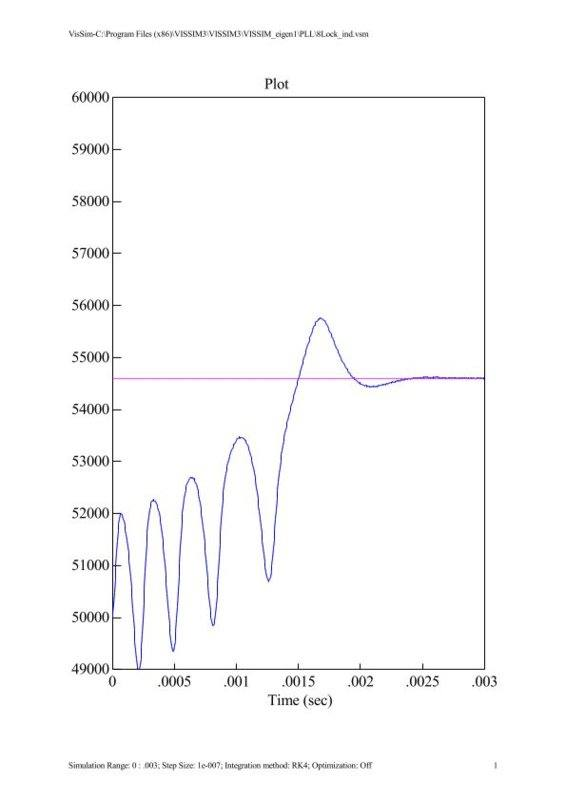Another use of PLLs is to decode a Frequency Shift Keyed (FSK) signal. This is a signal that encodes 1s and 0s as two different frequencies. For the VCO to track the two frequencies, its Control Voltage must change. The Control Voltage is the output of the Low Pass Filter. By detecting the control voltage, a circuit can output a High or Low voltage, thereby recovering the 1s and 0s. This is how Fax machines transmit an image over the telephone lines.
Maybe a bit more 'fresh' example would be optical or magnetic data storage. The encoded data comes as a stream, but with frequency/phase unknown (and it might even vary according to the accuracy of the spin motor control // drive strategy: hence the different maximal reading speed of CD/DVD rom drives when it is inner or outer areas of a disk). To recover the accurate frequency and phase of the reference clock relevant to the actual raw data stream is a job for a PLL.

•jaus tail
Thanks for all the replies. It's very helpful. Sorry for the late reply. I had gone home for holiday.

The goal of the control is to make the phase difference between input and output zero.

This is where I have doubt. If the difference between input and output phase is zero then the output of LPF will be zero and thus the VCO will get 0 signal in that case?
Or maybe the final stable has to be like there's a constant phase difference between two signals?

Baluncore
... the final stable has to be like there's a constant phase difference between two signals?
Correct.
The VCO will require a DC input voltage that will determine the VCO output frequency.
When locked, the low-pass filter will pass that DC component to the VCO control input.
There must be sufficient phase error to generate that average DC voltage.
A phase locked loop employs a phase comparison to lock the frequency.
It does not lock the phase to exactly 0° or 90°.

This is where I have doubt. If the difference between input and output phase is zero then the output of LPF will be zero and thus the VCO will get 0 signal in that case?
Or maybe the final stable has to be like there's a constant phase difference between two signals?

There is no general answer to this question - because it depends on the loop filter used. More than that, we have to distinguish between "static" and "dynamic" phase error.

1.) Static phase error (response to a phase step): Regarding the phase, the VCO works like an integrator (with memory !) and the static phase error will be zero (ideal case, with respect to the nominal phase difference, which depends on the phase detector used)

2.) Dynamic phase error (response to a phase ramp - identical to a frequency step):
The dynamic phase error depends on the loop filter used.
* Lag filter: Finite phase error (frequency step divided by the loop gain)
* PI Filter : Dyn. phase error will be zero (the integrating part of the filter works as a memory for the error signal to the VCO)

Last edited:
•jaus tail
Baluncore
The situation can be even more complex when the frequency inputs to the phase comparator do not have the same waveform.

•jaus tail
Oh boy! Electronics is super difficult. Electrical engineering is easier, to be frank, cause there at least we can visualize stuff. With electronics, it's more maths and equations :(

Oh boy! Electronics is super difficult. Electrical engineering is easier, to be frank, cause there at least we can visualize stuff. With electronics, it's more maths and equations :(

It gets easier with practice. :)

This is where I have doubt. If the difference between input and output phase is zero then the output of LPF will be zero and thus the VCO will get 0 signal in that case?
Or maybe the final stable has to be like there's a constant phase difference between two signals?

Tom G. called me out correctly because I was a bit sloppy with my terms. I should've said the goal of the control system is to make the error signal zero. For some PLL circuits, this happens when the input and the output have the exact same phase. But that's just the goal, in practice, they don't really have the exact same phase.

At risk of going off-topic, a PLL control system can be a bit difficult to analyze mathematically. To get the gist of control system errors perhaps try analyzing a simpler system. This app note  shows how to analyze some of the DC error of a basic opamp circuit. In particular, it shows how the finite gain of the control system causes DC error in the output. In a PLL the amount of DC gain would control how close to zero the error signal can get. There are even more sources of error than that but DC gain is an important one in many control systems.

 http://www.ti.com/lit/an/slyt374/slyt374.pdf